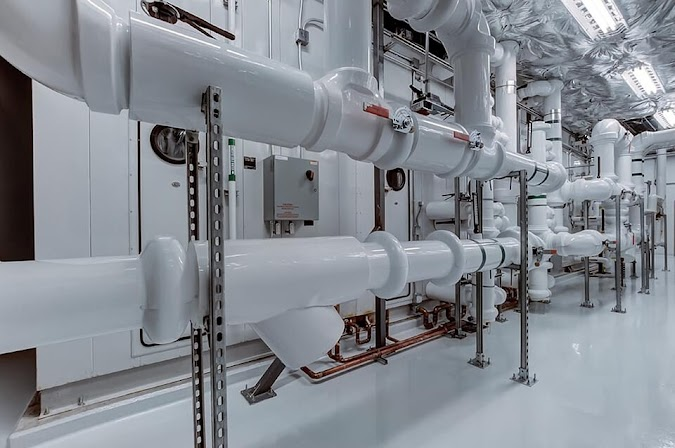What Is The Difference Between Rangeability And Turndown Ratio? | Mecholic -->

# What Is The Difference Between Rangeability And Turndown Ratio?Generally, the term rangeability and turndown ratio are associated the with control valves sizing and flow measuring devices. They often get confused and interchanged. Usually, it does not matter because both of them used to indicate the operational range of an instrument.

## Rangeability

Rangeability defined as the ratio of maximum controllable flow to the minimum controllable flow that a device designed to. So this is a theoretical value before a device installed.

## Turndown ratio

Turndown ratio gives the width of the operational range of a device/valve. It is not required to handle maximum possible flow. It defined as the ratio normal operating maximum system flow to the minimum controllable flow. In the case of boilers turndown ratio is the ratio of maximum heat output to the minimum level of heat output at which the boiler will operate controllably.

It is the characteristic of the device after the installation, and it is more of a process Parameter. The maximum value of turndown ratio is the rangeability

For example, an orifice meter allows maximum flow 6kg/s minimum flow 2kg/s; then range ability is 6/2 = 3/1.
In above example, if the normal maximum flow is 5kg/s; then turndown ratio is 5/2

Another example
If the minimum flow is 25% of maximum flow then the rangeability equal to 100/25 = 4.
If the valve required to handle normal maximum flow, that is 75% of maximum flow, then the turndown ratio is 75/25 = 3